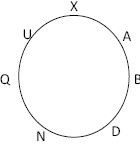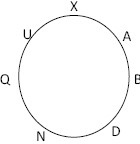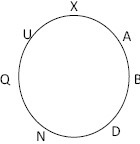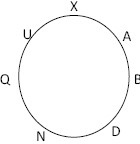# SBI Reasoning Question

DIRECTIONS for the question 1 to 4: Read the information given below and answer the question that follows.

Q, U, X, D, A, B and N are sitting around a circle facing at the center. D is third to the left of X who is second to the left of Q. U is third to the left of D and second to the right of A. B is not an immediate neighbour of Q.

1. Who is fourth to the right of Q?
1. D
2. B
3. X
4. U
5. None of aboveA is fourth to the right of Q.
2. Who among the following is second to the left of B?
1. N
2. Q
3. U
4. X
5. None of aboveN is second to the left of B
1. In which of the following pairs the second person is sitting to the immediate right of the first person?
1. XA
2. UX
3. BA
4. QU
5. None of the aboveA is sitting immediate right of B.
2. Who is third to the right of D?
1. A
2. X
3. Q
4. B
5. None of aboveX is third to the right of D
DIRECTIONS for the questions 5 - 8: Solve the following question and mark the most appropriate option.
3. If ‘A’ is substituted by 1, ‘B’ by 2 and so on upto ‘Z’, which is substituted by 26, what will be the sum of the numbers substituted for the word ‘SAVE’?
1. 36
2. 47
3. 50
4. 31
For word SAVE, S is 19, A is 1, V is 22, E is 5.
So adding the numbers, we get 19+1+22+5 = 47
4. There are thirty students in a class. Prakash ranks third among the boys. Priya ranks fifth among the girls. Prakash is one rank below Priya in the class. No two students hold the same rank. What is Priya’s rank in the class?
1. 5
2. 6
3. 7
4. 8
5. 9
Prakash ranks third among the boys. Priya ranks fifth among the girls.Prakash comes after Priya.
It means two boys and four girls rank higher than Priya in the class. Therefore, Priya ranks 7th in the class.
5. How many three letter meaningful words can be formed from the word REACTION beginning with ‘A’ and without repeating any letter within that word?
1. 6
2. 3
3. 4
4. 5
5. 2
Meaningful 3 letter words starting with ‘A’ are ACT, ACE, AIR, ANT, ATE, ART
6. Kanika’s birthday is on 27th January, 2012 i.e. Friday. Her friend, Sana’s birthday is exactly on the 5th Wednesday after her birthday. How much younger is Sana than Kanika?
1. 29 days
2. 30 days
3. 33 days
4. 32 days
5. 31 days
Kanika’s birthday is on 27th January, 2012. Now as per question, Sana’s birthday is on 29th February, 2012 ( Because 2012 is a leap year ). Therefore, Sana is 33 days younger than Kanika.
DIRECTIONS for questions 9 and 10 : What should come in place of question mark (?) in the following number/alphabetic series?
7. 3, 7, 13, 21, ?
1. 31
2. 34
3. 33
4. 35
5. 32